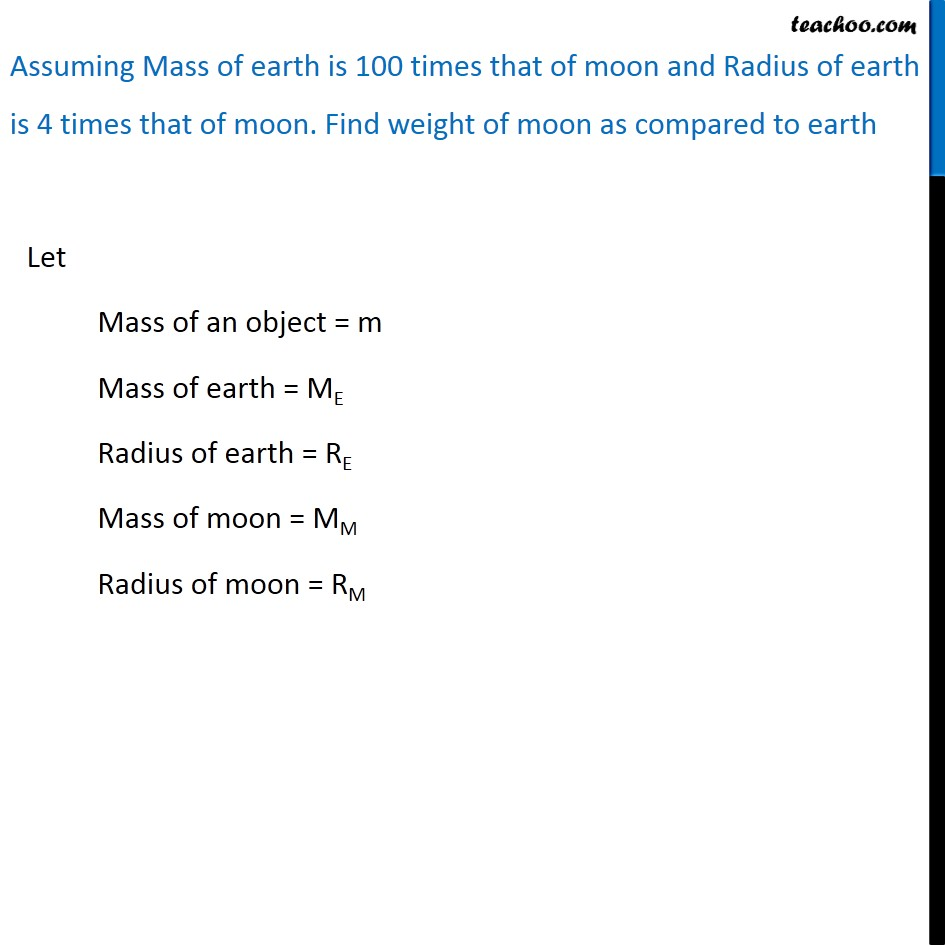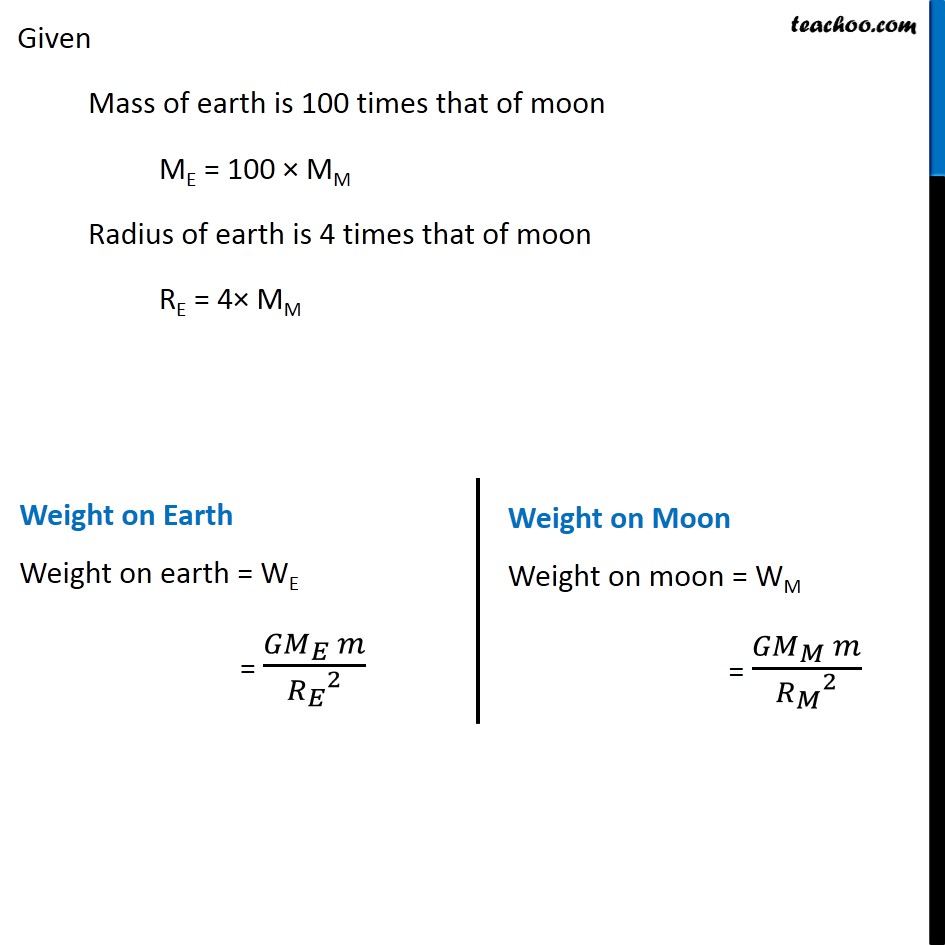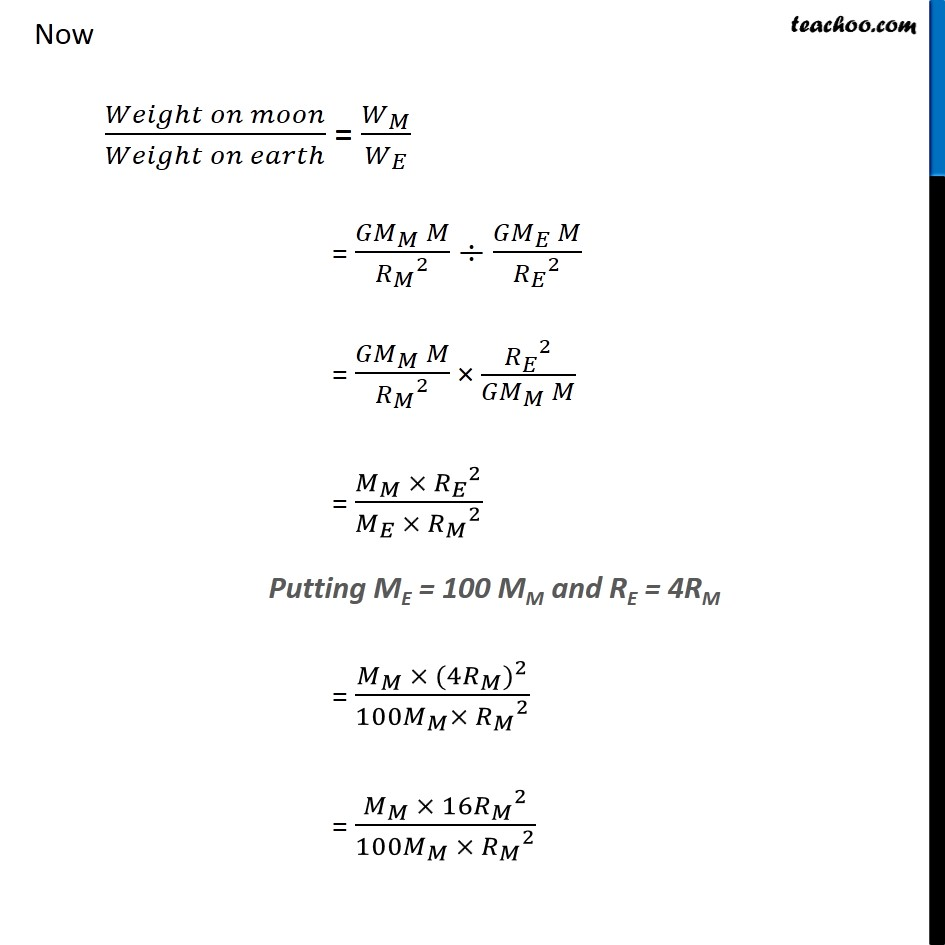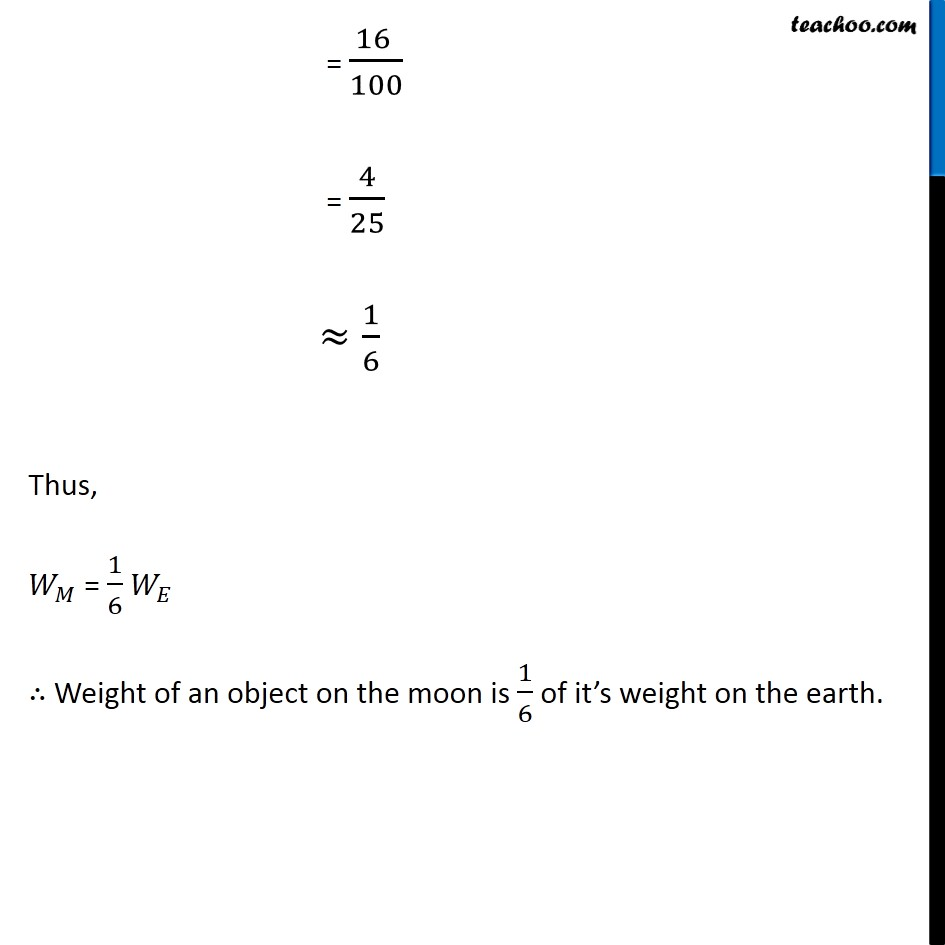Concepts

Class 9
Chapter 10 Class 9 - Gravitation

In general language, we use mass and weight interchangeably

However both are not same in scientific language

## Difference between Mass and Weight

 Mass Weight It is quantity of matter contained in a body It is a force by which a body is attracted towards center of earth It is measured in kilogram (SI Unit of mass is kg) It is measured in Newton (SI Unit of weight is N) Mass of a body remains constant Weight of an object changes as per acceleration due to gravity Mass of a body is same on earth and moon Weight of body on moon is 1/6 of mass of body on earth Mass of body can never be 0 In case acceleration due to gravity g is 0, weight can be 0 (example in space,weight of body is 0) Mass is a scalar quantity,it only has magnitude Weight is a vector quantity, it has magnitude as well as direction

## How is Weight of body calculated?

We know that

Force = Mass x Acceleration

Since Weight is also kind of a force by which a body is attracted towards center of earth,we can say that

Weight = Mass x Acceleration

In Place of Acceleration,we can use Acceleration due to gravity

Weight = Mass x Acceleration due to gravity

W = M x g

## What is weight of body of mass 1 kg?

We know that

W = m x g

In this case m = 1 kg, g = 9.8 m/s2

W = 1 x 9.8

W = 9.8

W = 9.8 Newton (kg m/s2)

## What is weight of body on moon

Mass of earth is 100 times that of moon

Radius of earth is 4 times that of moon

If we put these values in Gravitational Force formula,

We find that

Weight of a body on moon is 1/6th of weight of body on earth

Let's Prove itTo check exact proof, check Derivation

Learn in your speed, with individual attention - Teachoo Maths 1-on-1 Class

### Transcript

Assuming Mass of earth is 100 times that of moon and Radius of earth is 4 times that of moon. Find weight of moon as compared to earth Let Mass of an object = m Mass of earth = ME Radius of earth = RE Mass of moon = MM Radius of moon = RM Given Mass of earth is 100 times that of moon ME = 100 × MM Radius of earth is 4 times that of moon RE = 4× MM Weight on Earth Weight on earth = WE = (〖𝐺𝑀〗_𝐸 𝑚)/〖𝑅_𝐸〗^2 Weight on Moon Weight on moon = WM = (〖𝐺𝑀〗_𝑀 𝑚)/〖𝑅_𝑀〗^2 Now (𝑊𝑒𝑖𝑔ℎ𝑡 𝑜𝑛 𝑚𝑜𝑜𝑛)/(𝑊𝑒𝑖𝑔ℎ𝑡 𝑜𝑛 𝑒𝑎𝑟𝑡ℎ) = 𝑊_𝑀/𝑊_𝐸 = (〖𝐺𝑀〗_𝑀 𝑀)/〖𝑅_𝑀〗^2 ÷ (〖𝐺𝑀〗_𝐸 𝑀)/〖𝑅_𝐸〗^2 = (〖𝐺𝑀〗_𝑀 𝑀)/〖𝑅_𝑀〗^2 × 〖𝑅_𝐸〗^2/(〖𝐺𝑀〗_𝑀 𝑀) = (𝑀_𝑀 × 〖𝑅_𝐸〗^2)/(𝑀_𝐸 × 〖𝑅_𝑀〗^2 ) Putting ME = 100 MM and RE = 4RM = (𝑀_𝑀 × 〖(4𝑅_𝑀)〗^2)/(100𝑀_𝑀× 〖𝑅_𝑀〗^2 ) = (𝑀_𝑀 × 16〖𝑅_𝑀〗^2)/(100𝑀_𝑀 × 〖𝑅_𝑀〗^2 ) = (16 )/100 = 4/25 ≈1/6 Thus, 𝑊_𝑀 = 1/6 𝑊_𝐸 ∴ Weight of an object on the moon is 1/6 of it’s weight on the earth.